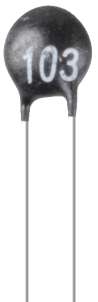# Thermistor¶A thermistor is a type of resistor whose resistance is strongly dependent on temperature, more so than in standard resistors. The word is a combination of thermal and resistor. Thermistors are widely used as inrush current limiters, temperature sensors (negative temperature coefficient or NTC type typically), self-resetting overcurrent protectors, and self-regulating heating elements (positive temperature coefficient or PTC type typically).

Here is the electronic symbol of thermistor.Thermistors are of two opposite fundamental types:

• With NTC thermistors, resistance decreases as temperature rises usually due to an increase in conduction electrons bumped up by thermal agitation from valency band. An NTC is commonly used as a temperature sensor, or in series with a circuit as an inrush current limiter.

• With PTC thermistors, resistance increases as temperature rises usually due to increased thermal lattice agitations particularly those of impurities and imperfections. PTC thermistors are commonly installed in series with a circuit, and used to protect against overcurrent conditions, as resettable fuses.

In this kit we use an NTC one. Each thermistor has a normal resistance. Here it is 10k ohm, which is measured under 25 degree Celsius.

Here is the relation between the resistance and temperature:

RT = RN * expB(1/TK - 1/TN)

• RT is the resistance of the NTC thermistor when the temperature is TK.

• RN is the resistance of the NTC thermistor under the rated temperature TN. Here, the numerical value of RN is 10k.

• TK is a Kelvin temperature and the unit is K. Here, the numerical value of TK is 273.15 + degree Celsius.

• TN is a rated Kelvin temperature; the unit is K too. Here, the numerical value of TN is 273.15+25.

• And B(beta), the material constant of NTC thermistor, is also called heat sensitivity index with a numerical value 3950.

• exp is the abbreviation of exponential, and the base number e is a natural number and equals 2.7 approximately.

Convert this formula TK=1/(ln(RT/RN)/B+1/TN) to get Kelvin temperature that minus 273.15 equals degree Celsius.

This relation is an empirical formula. It is accurate only when the temperature and resistance are within the effective range.

Example